Reach Us+44 1704 335730
Solution of Voltra-Fredholm Integro-Differential Equations using Chebyshev Collocation Method | OMICS International
Global Journal of Technology and Optimization
All submissions of the EM system will be redirected to Online Manuscript Submission System. Authors are requested to submit articles directly to Online Manuscript Submission System of respective journal.

# Solution of Voltra-Fredholm Integro-Differential Equations using Chebyshev Collocation Method

Deepmala1, Vishnu Narayan Mishra2*, HR Marasi3, H Shabanian4 and M Nosrati Sahlan4

1Mathematics Discipline, PDPM Indian Institute of Information Technology, Design and Manufacturing, Jabalpur-482005, India

2Applied Mathematics and Humanities Department, S.V. National Institute of Technology, Surat-395007, Gujarat, India

3Department of Applied Mathematics, Faculty of Mathematical Sciences, University of Tabriz, Tabriz, Iran

4Department of Mathematics, Faculty of Sciences, University of Bonab, Bonab, Iran

*Corresponding Author:
Vishnu Narayan Mishra
Applied Mathematics and Humanities Department
S.V. National Institute of Technology
Surat-395007, Gujarat, India
Tel: +91 99133 87604
E-mail: [email protected]

Received date: February 23, 2017; Accepted date: April 20, 2017; Published date: April 26, 2017

Citation: Deepmala, Mishra VN, Marasi H, Shabanian H, Nosrati Sahlan M (2017) Solution of Voltra-Fredholm Integro-Differential Equations using Chebyshev Collocation Method. Global J Technol Optim 8:210. doi: 10.4172/2229-8711.1000210

Copyright: © 2017 Deepmala, et al. This is an open-access article distributedunder the terms of the Creative Commons Attribution License, which permits unrestricted use, distribution, and reproduction in any medium, provided the original author and source are credited.

Visit for more related articles at Global Journal of Technology and Optimization

#### Abstract

In this paper, we use chebyshev polynomial basis functions to solve the Fredholm and Volterra integro-differential equations. We directly calculate integrals and other terms are calculated by approximating the functions with the Chebyshev polynomials. This method affects the computational aspect of the numerical calculations. Application of the method on some examples show its accuracy and efficiency.

#### Keywords

Integro-differential equation; Chebyshev polynomial; Collocation method

#### Introduction

We consider the integro-differential equations of Fredholm, Volterra and Fredholm-Volterra types in the forms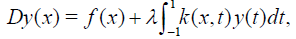(1)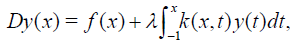(2)

and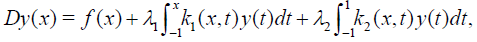(3)

where λ, λ1, and λ2 are real parameters. The functions f(x), k(x,t), k1(x,t) and k2(x,t) are known, y(x) is the unknown function to be determined and D is a linear differential operator. We suppose, without loss of generality, that the interval of integration is [-1,1]. Many problems in engineering and mechanics can be transformed into integral equations. For example it is usually required to solve Fredholm integral equations(FIE) in the calculations of plasma physics . The numerical solution of these equations is a well-studied problem and a large variety of numerical methods have been developed to rapidly and accurately obtain approximations to y(x). Overviews and references to the literature for many existing methods are available in [2,3]. Collocation methods [2-6], Sinc methods , global spectral methods , methods for convolution equations , Newton-Gregory methods , Runge-Kutta methods [11,12], qualocation methods  and Galerkin methods  are several of the many approaches that have previously been considered. In this paper the aim is to obtain the solution as a truncated Chebyshev series defined by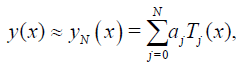(4)

where Tj(x) denotes the Chebyshev polynomials of the frist kind, aj are unknown Chebyshev coefficients and N is any chosen positive integer. The Chebyshev collocation points are defined by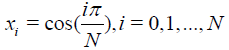(5)

The paper is organized as follows: In Section Approximations we describe numerical approximations for differential operator and functions of integro-differential equation. The numerical results are presented in Section Numerical examples.

#### Methods and Approximations

Let D be a linear differential operator of order v with polynomial coefficients defined by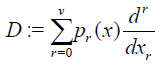(6)

We shall write for pr (x)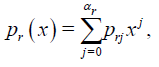(7)

Where αr is the degree of pr(x).

Let y(x) be the exact solution of the integro-differential equation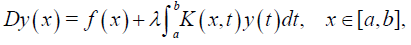(8)

with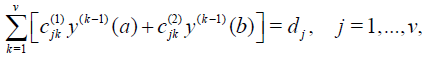(9)

Where f(x) and k(x,t) are given continuous functions and λ, a, b,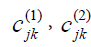and dj some given constants.

Matrix representation for Dy(x)

Let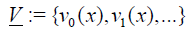be a polynomial basis given by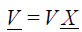where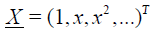and V is a non-singular lower triangular matrix with degree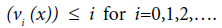According to  the effect of differentiation, shifting and integration on the coefficients vectorof a polynomial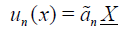is the same as that of post-multiplication of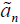by the matrices Æ�, rμ and l respectively,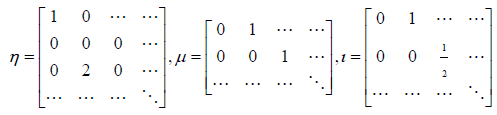Where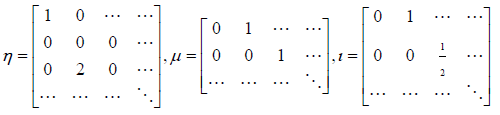We recall now the following theorem given by Oritez and Samara .

Theorem 4.1.1 For any linear differential operator D defined by Eq. (6) and any series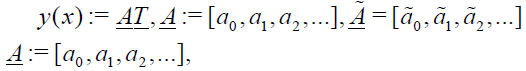we have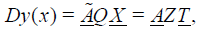where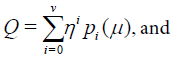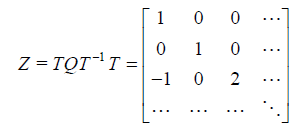Function approximation

The solution of Eqs. (1), (2) and (3) can be expressed as a truncated Chebyshev series. Therefore, the approximate solution (4) can be written in the matrix from

y (x) = TT (x)A, (10)

where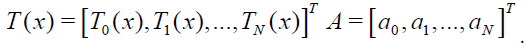Consequently, using Theorem 2.1 and substituting Eq. (10) in Eq. (1), we get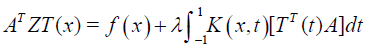(11)

Now using the chebyshev collocation points (5) in Eq. (11) we obtain the following new system of algebraic equations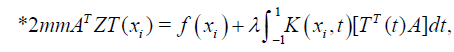(12)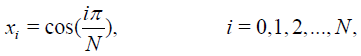and so, unknown coefficients aj are found.

Definition 4.2.1 The polynomial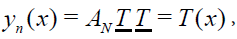will be called an approximate solustion of Eqs. (8) and (9), if the vector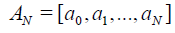is the solution of the system of liner algebraic equations (12).

Similarly we can develop the method for the problem defined in the domain [0, 1]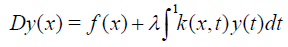In this case we obtain the solution in terms of shifted Chebyshev polynomials T*j (x) in the form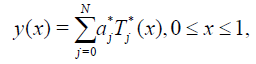where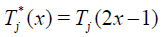Similar to the previous procedure and using the collocation points defined by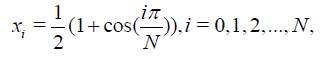(13)

one can get the following system of algebraic equations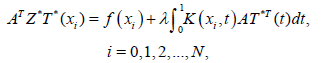(14)

where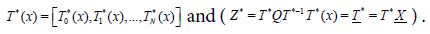Solving the nonlinear system, unknown coefficients aj are found. Similarly, we obtain the fundamental equation for Volterra and Fredholm-Volterra integral equation. In this study, instead of approximating integral terms, we directly calculate integrals. Examples show that this method affects the computational aspect of the numerical calculations.

#### Results and Numerical Examples

The results obtained in previous sections are used to introduce a direct efficient and simple method to solve integro-differential equations of Volterra and Fredholm type.

Example 5.1 We consider the following Fredholm integrodifferential equation of the second kind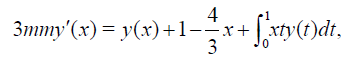y(0)=0,3mm

The exact solution is y(x) =cx. We assume the solution of y(x) as a truncated Chebyshev series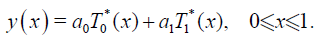(15)

Here, we have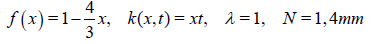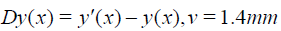The fundamental equation of the problem is defined by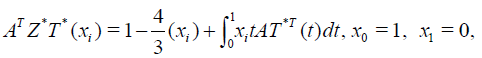where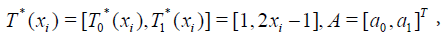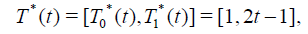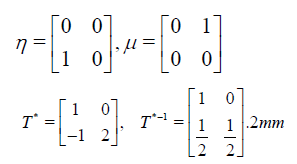Therefore, using Theorem 4.1.1 we obtain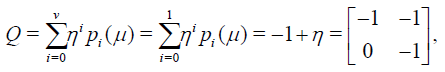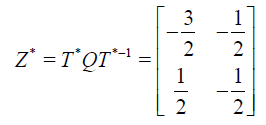The system yields the solution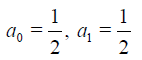Substituting these values in (15), we get the exact solution of the problem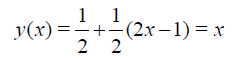Example 5.2 We consider the following Fredholm-Volterra integrodifferential equation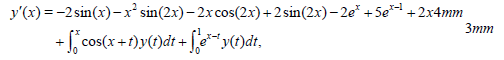y(0) = 0,3mm

The exact solution is y(x) = x2 . Let us suppose that y(x) is approximated by Chebyshev series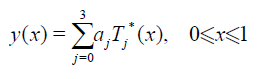Using the procedure in section Approximations, we obtain the approximate solution of the problem.

In Table 1, we compare the numerical results of the problem by the proposed method of N=3 with the method discussed in an earlier study .

X The method discussed in  Presented method N=3
0 4.930 × 10-4 3.3590 × 10-15
0.1 2.240 × 10-3 3.7764 × 10-15
0.2 1.571 × 10-3 4.2889 × 10-15
0.3 1.514 × 10-3 4.8711 × 10-15
0.4 7.015 × 10-3 5.5788 × 10-15
0.5 1.6336 × 10-2 6.2727 × 10-15
0.6 1.1862 × 10-2 7.0499 × 10-15
0.7 4.971 × 10-3 7.8825 × 10-15
0.8 4.338 × 10-3 8.9928 × 10-15
0.9 1.6068 × 10-2 9.8809 × 10-15
1.0 ---- 1.0880 × 10-15

Table 1: Comparison of the absolute errors of example (3.2).

Example 5.3 We consider the following Fredholm integro-differential equation of the second kind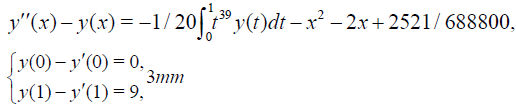The exact solution is y(x) = x2 + 2x + 2 . Talking N = 2,4, the approximate solutions are obtained by this method. Results are compared with those of the methods in literature as shown in Table 2.

x Method in  presented method N=2 presented method N=4
0 0.0187621362 0.0036921151 0.002472602103
1/15 0.0200637354 0.0036087818 0.002472602236
2/15 0.0212780889 0.0035254484 0.002472602365
3/15 0.0215334191 0.0034421151 0.002472602492
4/15 0.0205212581 0.0033587818 0.002472602617
5/15 0.0192905931 0.0032754484 0.002472602740
6/15 0.0181294338 0.0031921151 0.002472602862
7/15 0.0170353464 0.0031087818 0.002472602984
8/15 0.0160143431 0.0030254484 0.002472603106
9/15 0.0150618428 0.0029421151 0.002472603229
10/15 0.0141627843 0.0028587818 0.002472603353
11/15 0.0133104506 0.0027754484 0.002472603479
12/15 0.0125010566 0.0026924451 0.002472603607
13/15 0.0117309887 0.0026087818 0.002472603788
14/15 0.0109968073 0.0025254484 0.002472603873
1.0 0.0102952225 0.0024421151 0.002472604012

Table 2: Comparison of the absolute errors of example (3.3).

Example 5.4 We consider the following Volterra integro-differential equation of the second kind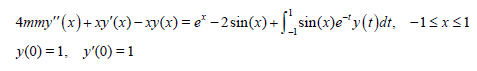The exact solution is y(x) = ex . See Table 3 for the numerical results.

X Exact Presented method N=11
-1 0.36787944 0.36787445212
-0.8 0.44932896 0.44932342567
-0.6 0.54881164 0.54881194564
-0.4 0.67032005 0.67032187409
-0.2 0.81873075 0.81897235137
0 1 0.99988319565
0.2 1.22140276 1.22140675944
0.4 1.49182470 1.49189543017
0.6 1.82211880 1.82211675430
0.8 2.22554093 2.22554075420
1.0 2.71828183 2.71828147693

Table 3: Comparison of numerical results for example (3.4).

#### References

Select your language of interest to view the total content in your interested language

### Article Usage

• Total views: 1169
• [From(publication date):
April-2017 - Sep 15, 2019]
• Breakdown by view type
• HTML page views : 1024

## Post your commentCan't read the image? click here to refresh
###### Peer Reviewed Journals

Make the best use of Scientific Research and information from our 700 + peer reviewed, Open Access Journals

International Conferences 2019-20

Meet Inspiring Speakers and Experts at our 3000+ Global Annual Meetings

Top